# Identifying Duplicate Questions: A Machine Learning Case Study

Source: Deep Learning on MediumPhoto by Matthew Henry on Unsplash

This blog post is adapted from a capstone project I created for the Data Science Career Track at Springboard.

### PROBLEM

Quora and Stack Exchange are knowledge-sharing platforms where people can ask questions in the hopes of attracting high-quality answers. Often, questions that people submit have previously been asked. Companies like Quora can improve user experience by identifying these duplicate entries. This would enable users to find questions that have already been answered and prevent community members from answering the same question multiple times.

Consider the following pair of questions:

1. Is talent nurture or nature?
2. Are people talented by birth or can it be developed?

These are duplicates; they are worded differently, but they have the same intent. This blog post focuses on solving the problem of duplicate question identification.

### SOLUTION

Suppose we have a fairly large data set of question-pairs that has been labeled (by humans) as “duplicate” or “not duplicate.” We could then use natural language processing (NLP) techniques to extract the difference in meaning or intent of each question-pair, use machine learning (ML) to learn from the human-labeled data, and predict whether a new pair of questions is duplicate or not.

This post explores a few of these NLP and ML techniques, like text pre-processing, embedding, logistic regression, gradient-boosted machine, and neural networks. The general approach of the solution is outlined in this high-level diagram: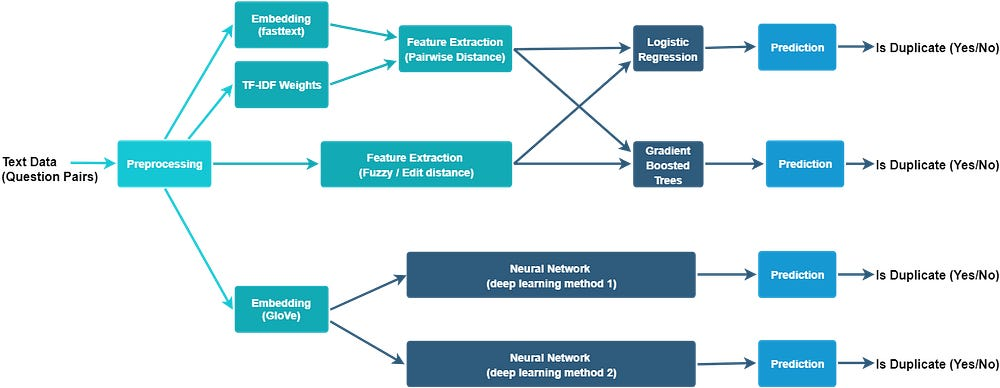Overview of NLP and classification techniques explored in this blog post

### DATA

The data, from Kaggle (Quora Question Pairs), contains a human-labeled training set and a test set. Each record in the training set represents a pair of questions and a binary label indicating whether it’s a duplicate or not.Sample from the data set

### TEXT PRE-PROCESSING

Text data typically requires some cleanup before it can be embedded in vector space and fed to a machine learning model. The question data can be cleaned by removing elements that don’t make a significant contribution to their meaning — like tags, repeating whitespace, and frequently-occurring words — and by transforming to an easily parseable format as described in the steps and code snippet below:

1. Remove tags. For example, “<i>Hello</i> <b>World</b>!” is converted to “Hello World!”
2. Remove repeating whitespace characters (spaces, tabs, line breaks). Convert tabs and line breaks to spaces.
3. Remove stopwords. These include the most commonly occurring words in a language, like “the,” “on,” “is,” etc. NLP libraries like gensim provide a default list of stopwords.
4. Convert all text to lowercase.
5. Perform Porter stemming. Porter stemming reduces inflections like “fishing,” “fished,” and “fisher” to the root “fish.” This makes it easier for an ML model to learn how to glean meaning or intent form a sequence of words.Text pre-processing with gensim

### EMBEDDING — TEXT TO NUMBERS

Embedding maps words in a text document to number space (these number-space representations are called word vectors). Word embeddings require extremely large data sets for effective training. Often, embeddings that are pre-trained on large text data sets like Wikipedia and Common Crawl are used successfully to solve NLP problems that may not have a big enough data set for training the embedding.

This post explores two different methods to embed the text data in vector space:

Fasttext — For the first two models (logistic regression and gradient-boosted machine), we’ll use Fasttext embedding. The Fasttext model for English is pre-trained on Common Crawl and Wikipedia text.

Fasttext represents each word as a set of sub-words or character n-grams. For example, the word “fishing” is represented, assuming a subword length of 3 (trigram), as follows:

`{‘fishing’, ‘fis’, ‘ish’, ‘shi’, ‘hin’, ‘ing’}`

Character n-gram representation enables more robust handling of Out Of Vocabulary (OOV) words than other embedding methods like word2vec.

To combine the benefits of training on large data sets like Wikipedia as well as on problem-specific training data, the pre-trained model can be further trained on questions in the training data, using the technique of transfer-learning. The gensim method intersect_word2vec_format can be used for transfer-learning. This method initializes a word2vec model with the vocabulary of the training data, then intersects this vocabulary with the pre-trained model (see code snippet below). No words are added to the existing vocabulary, but intersecting words adopt the weights of the pre-trained model, while non-intersecting words are left alone.Transfer learning of pre-trained fasttext model using gensim

GloVe — For the next two models (deep learning), the Spacy model for English will be used for embedding. This model is pre-trained on Common Crawl using GloVe. A provision can be made for OOV words by randomly mapping each OOV word to one of 50 randomly generated vectors (see code below).Embedding with Spacy and handling OOV words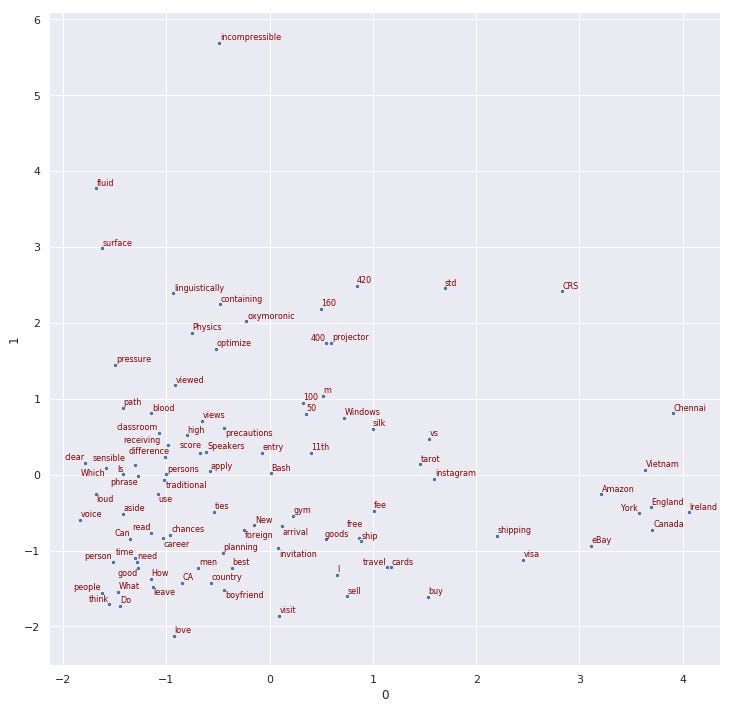The plot above shows word-vectors for 20 questions sampled from the Quora data set. Dimension reduction was used to reduce the vectors from 300 to two dimensions. There’s some clustering based on meaning/semantics (e.g., names of places are bunched together on the bottom right of the plot)

### FEATURE ENGINEERING

The next step in the process after preprocessing and embedding the question-pair data is feature extraction. We’ll extract three different sets of features:

#### FEATURE SET 1 — SIMILARITY USING PAIRWISE DISTANCE

The similarity between questions can be computed using word-to-word (pairwise) distances, which are weighted with TF-IDF weights and then averaged over all word-pairs across a pair of questions (see Fig 2 below).

Pairwise Distances — We can compute the pairwise distances for each pair of words by picking the first word(t1) from question 1 and the second word(t2) from question 2 (step 1 in Fig 2).

Several pairwise distance metrics can be used, including Chebyshev, Bray-Curtis, Cosine, Correlation, Canberra, Cityblock, Euclidean, L1, L2, Minkowski, Squared Euclidean, and Hausdorff. Fig 1 below shows a comparison of cosine and Euclidean distances.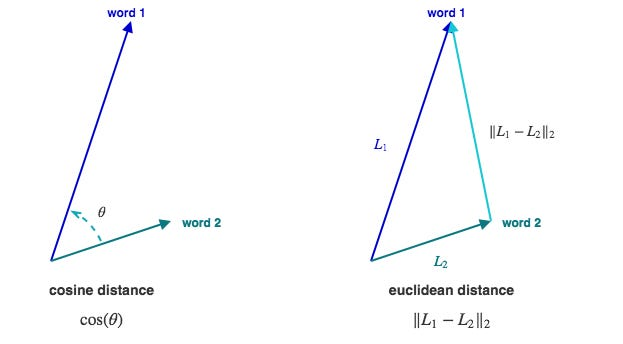Fig 1 — Geometric illustration of cosine and Euclidean distances in two dimensions

TF-IDF Weights — Term Frequency-Inverse Document Frequency is a weighting statistic used in many NLP applications. The value of this statistic increases proportionally with the number of times a word appears in a question and decreases proportionally with the total number of questions in the entire question-pair data set that contain that word. Used as a weighting factor, this statistic has the effect of downplaying words that are frequent across the entire vocabulary and highlighting the contribution of words that are frequent only in a specific question, thereby enabling better capture of semantics or meaning.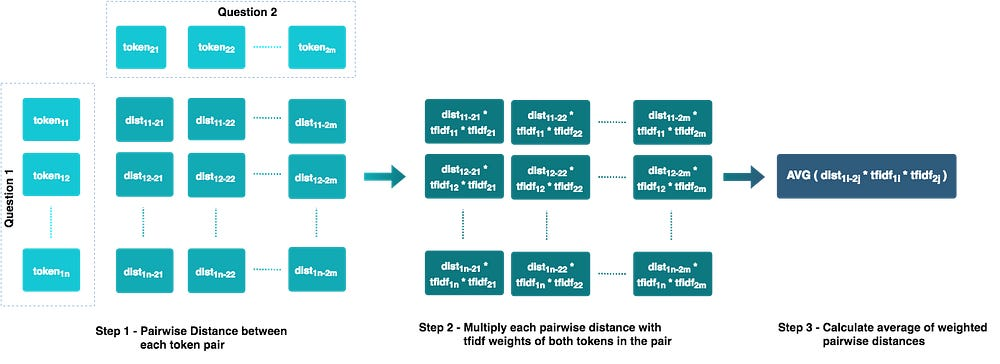Fig 2 — Steps used to compute the mean distance/similarity between sentences for feature set 1TF-IDF weights computationFeature computation using the weighted average of pairwise distance metrics

#### FEATURE SET 2 — SIMILARITY USING LEVENSHTEIN DISTANCE

Levenshtein Distance measures the difference between two text sequences based on the number of single character edits (insertions, deletions, and substitutions) it takes to change one sequence to another. It is also known as “edit distance.”

The Python library fuzzy-wuzzy can be used to compute the following metrics from the preprocessed data (the examples are from the fuzzy-wuzzy blog):

Simple Ratio — This computes the similarity between two word-sequences (in this case, the two questions) using the simple edit distance between them.

``fuzz.ratio("YANKEES", "NEW YORK YANKEES") ⇒ 60fuzz.ratio("NEW YORK METS", "NEW YORK YANKEES") ⇒ 75``

Partial Ratio — This improves on the simple ratio method above using a heuristic called “best partial,” which is useful when the two sequences are of noticeably different lengths. If the shorter sequence is length m, the simple ratio score of the best matching substring of length m is taken into account.

``fuzz.partial_ratio("YANKEES", "NEW YORK YANKEES") ⇒ 100fuzz.partial_ratio("NEW YORK METS", "NEW YORK YANKEES") ⇒ 69``

Token Sort Ratio — This involves tokenizing each sequence, sorting the tokens alphabetically, and then joining them back. These new sequences are then compared using the simple ratio method.

``fuzz.token_sort_ratio("New York Mets vs Atlanta Braves", "Atlanta Braves vs New York Mets") ⇒ 100``

Token Set Ratio — This involves tokenizing both the sequences and splitting the tokens into three groups: the intersection component common to both sequences and the two remaining components from each sequence. The scores increase when the intersection component makes up a larger percentage of the full sequence. The score also increases with the similarity of the two remaining components.

``t0 = "angels mariners"t1 = "angels mariners vs"t2 = "angels mariners anaheim angeles at los of seattle"fuzz.ratio(t0, t1) ⇒ 90fuzz.ratio(t0, t2) ⇒ 46fuzz.ratio(t1, t2) ⇒ 50fuzz.token_set_ratio("mariners vs angels", "los angeles angels of anaheim at seattle mariners") ⇒ 90``Computation of Levenstein distance metrics

#### FEATURE SET 3 — WORD VECTORS

Each question can be converted into a sequence of word-vectors using pre-trained embeddings that are then averaged with TF-IDF weights to find the “weighted centroid” representation of each question.Computation of weighted centroids of the word-vector sequence for each question

#### FEATURE COMPARISON

The plots below give a qualitative idea about how hand-engineered features (sets 1 and 2) might contribute to the classification. It is obvious from these plots that the hand-engineered features are beginning to separate the duplicates from non-duplicates, but are not good enough by themselves and need help from features in set 3 (embedding vectors).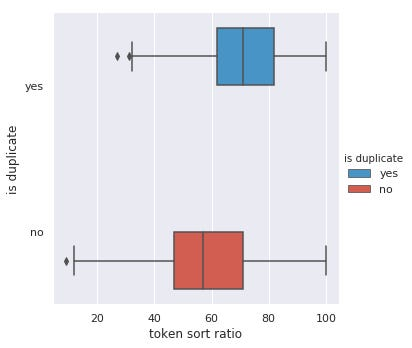Box plots show the distribution for the Levenshtein distances token-sort-ratio and token-set-ratio and the Hausdorff pairwise distance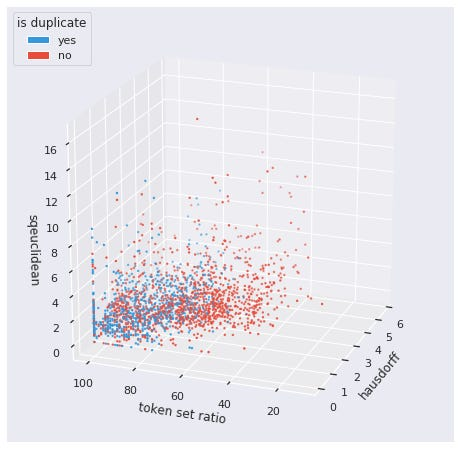A 3D scatter plot shows how duplicate (blue) and non-duplicate (red) question-pairs vary with square Euclidean distance, token sort ratio, and Hausdorff distance

### SPLITTING DATA INTO TRAINING, VALIDATION, AND TEST SETS

The question-pair data set must be split into training and test sets using a suitable train-test ratio (a good ratio for this data set is 2:1).

For deep learning, the training data can be further split into training and validation sets (a good ratio to use is 4:1). The validation data is used to evaluate the model during tuning (optimization). For logistic regression and gradient boosted machine, n-fold cross-validation can be used for tuning. A good value of n is five or 10.

### MODELING WITH LOGISTIC REGRESSION

Logistic regression is a linear model for classification. In this model, the probabilities describing the possible outcomes of a single trial are modeled using a logistic function. The logistic function is a sigmoid function, which takes any real input and outputs a value between 0 and 1, and hence is ideal for classification.

When a model learns the training data too closely, it fails to fit new data or predict unseen observations reliably. This condition is called overfitting and is countered, in one of many ways, with ridge (L2) regularization. Ridge regularization penalizes model predictors if they are too big, thus enforcing them to be small. This reduces model variance and avoids overfitting.

#### Hyperparameter Tuning

Cross-validation is a good technique to tune model parameters like regularization factor and the tolerance for stopping criteria (for determining when to stop training). Here, a validation set is held out from the training data for each run (called fold) while the model is trained on the remaining training data and then evaluated on the validation set. This is repeated for the total number of folds (say five or 10) and the parameters from the fold with the best evaluation score are used as the optimum parameters for the model.Model tuning, training, and prediction for logistic regression

#### Model Evaluation

The tuned model is first trained on the training data and then evaluated on the test data. It would be necessary to keep false positives (non-duplicates that have been incorrectly classified as duplicates) at a minimum as we do not want questions to be tagged with incorrect answers. This requires a model with high precision. Accuracy and the area under the receiver operating curve (ROC) are also useful metrics.

The following evaluation metrics were computed for logistic regression on the test data:

`accuracy ............... 0.75`
`precision .............. 0.68`
`recall ................. 0.61`
`area-under-roc-curve ... 0.72`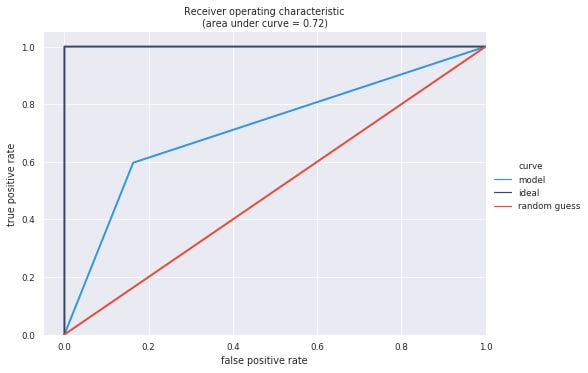Receiver operating characteristic curve for logistic regression

### MODELING WITH GRADIENT BOOSTED MACHINE

XGBoost (extreme gradient boosting) finds an optimum ensemble (combination) of several decision trees. It is an implementation of the gradient boosting technique introduced in the paper Greedy Function Approximation: A Gradient Boosting Machine, by Jerome H. Friedman. It can be considered a special case of gradient descent (in functional space), where lowering the loss function at each step of the descent enables the model to perform the next optimum decision split.

Overfitting is countered by limiting the depth of the component trees and by applying L2 regularization to the leaf-weights of the trees.

#### Hyperparameter Tuning

N-fold cross-validation (where n is five or 10) is once again used to tune model parameters, some of which are:

1. n_estimators (number of boosted trees to fit)
2. reg_lambda (L2 regularization term on weights)
3. max_depth (maximum tree depth for base learners)
4. learning_rate (boosting learning rate (xgb’s “eta”))
5. gamma (minimum loss to make a partition on a leaf)Model tuning, training, and prediction for gradient boosted machine

#### Model Evaluation

The following evaluation metrics were computed for the GBM using the test data. It is immediately evident that GBM performs far better than logistic regression in this case.

`accuracy ............... 0.84`
`precision .............. 0.76`
`recall ................. 0.79`
`area-under-roc-curve ... 0.81`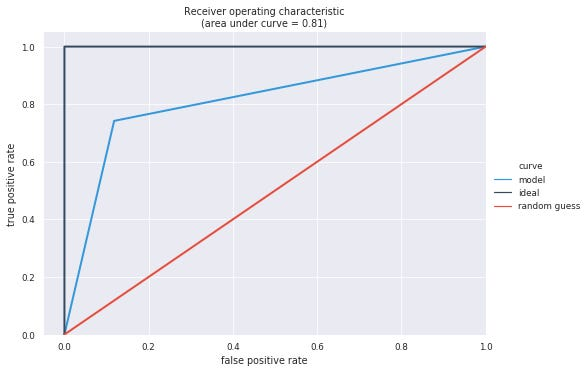Receiver operating characteristic curve for gradient boosted machine

### DEEP LEARNING WITH DECOMPOSABLE ATTENTION

This method is adapted from Example 1: A Decomposable Attention Model for Natural Language Inference from a blog post by Matthew Honnibal. The post implements the algorithm from the paper A Decomposable Attention Model for Natural Language Inference by Ankur P. Parikh et al. The paper uses attention to tackle the problem of predicting a class label over pairs of sentences, where the class represents the logical relationship between them.

Attention literally means “to pay attention” to each word in one question while encoding every word in the other question. The encoding is done by computing dot products of the word-vectors of the first question with the vectors of the second one.

A crucial advantage is provided by the fact that sentence-to-vector reduction operates over the pair of sentences jointly, as opposed to encoding the sentences into vectors independently. This captures the relationship between the two questions in a pair.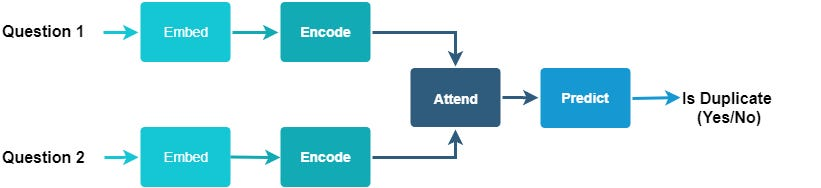High-level components of the neural network. The encode layer performs soft alignment with tokens in the other question to compute attention weights for the subsequent attention layer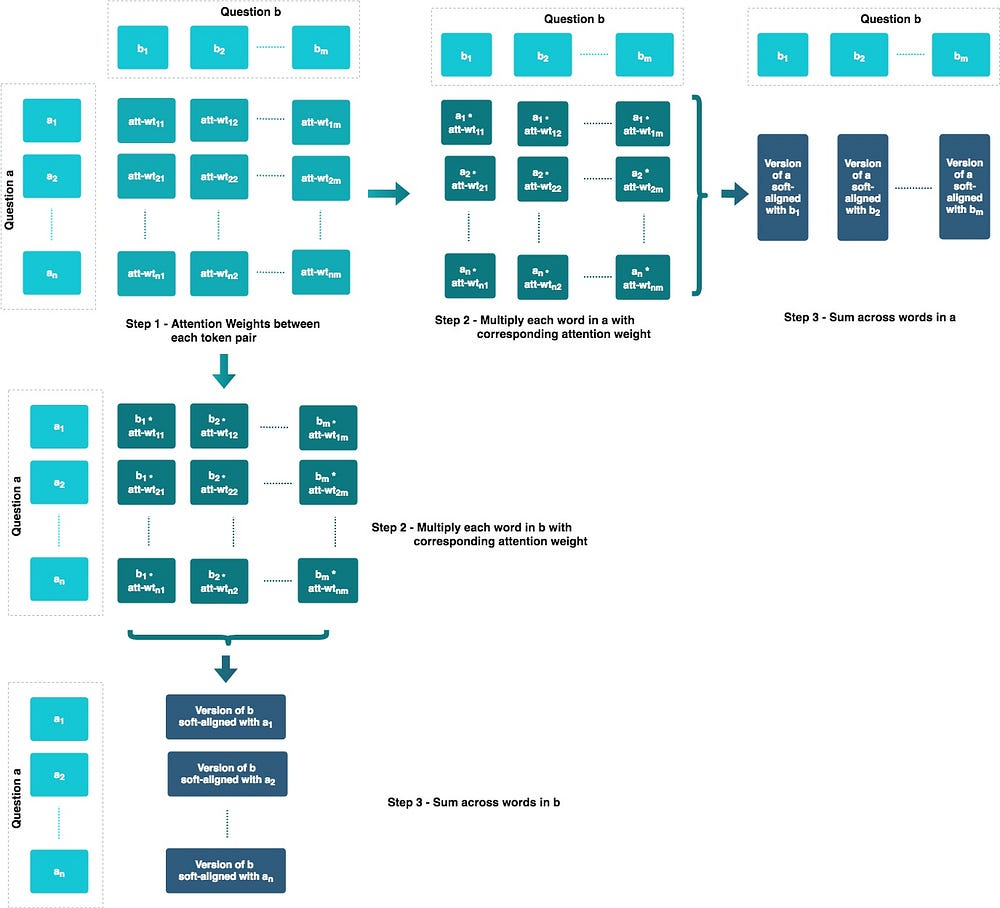Low-level detail of the embed step to illustrate the soft-alignment

The layers of the model are:

1. Embed — Converts tokens from text to vector space.
2. Encode — Computes weights using the dot product (pairwise cosine distance) of the vectors of question a with the vectors of question b (step 1 in the figure above). Next, the soft-aligned version of each question is computed with reference to every word in the other question (steps 2 and 3 in the figure above). This step uses a feed-forward layer (F) to compute the attention weights.
3. Attend — Combines the soft-aligned vectors with the corresponding word vectors and reduces them to a single vector per question. This step uses a feed-forward layer (G) to combine the soft-aligned vectors with the corresponding word vectors.
4. Predict / Classify — This step uses the final feed-forward layer (H) for predicting the output.

Overfitting is countered with dropout regularization and early stopping (see hyperparameter tuning below for early stopping). Dropout, performed for every feed-forward layer, tackles overfitting by randomly “dropping out” or ignoring neurons during training.Functions to create the decomposable attention model using Keras

#### Hyperparameter Tuning

The hyperparameters of the model are:

1. Dropout regularization rate for network F
2. Dropout regularization rate for network G
3. Dropout regularization rate for network H
4. Optimization method (Adam / RMSProp / Nadam / SGD )
5. Learning rate for the optimization method
6. Number of samples (batch size) per gradient update
7. The numbers of epochs used for training

The model parameters can be optimized using a sequential optimization technique like tree-structured Parzen estimator (TPE). TPE is used for efficiently searching in high-dimensional spaces. This is a sequential model-based optimization (SMBO) method, which builds and evaluates models sequentially, based on historic values of hyperparameters. The Python library hyperas, which implements TPE, can be used for optimization.

In this case, we conduct two search runs, one coarse-grained and the other fine-grained. The coarse-grained search determines the batch-size and the fine-grained search determines optimum values for the remaining parameters. The plot below tracks the variation of training and validation accuracy during the fine-grained search. There are several instances where the model overfits (points at which validation accuracy is significantly lower than the training accuracy).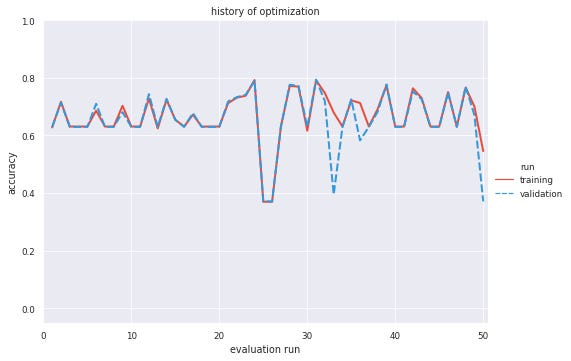The variation of training and validation accuracy during the fine-grained hyperparameter search (50 evaluations)

Early stopping stops training when a given validation metric stops improving (therefore stopping when the model begins to overfit). The Keras implementation of early stopping can be used during the hyperparameter search process to prevent the search algorithm from wasting time exploring local minima. This is implemented in the form of a callback that stops training if there is no improvement in the objective function.Tuning the decomposable attention model using Hyperas

#### Model Evaluation

The following evaluation metrics were computed for the decomposable attention model using the test data. Gradient boosted machine is still a better model than decomposable attention in terms of accuracy and area under the ROC curve.

`accuracy ............... 0.80`
`precision .............. 0.76`
`recall ................. 0.72`
`area-under-roc-curve ... 0.77`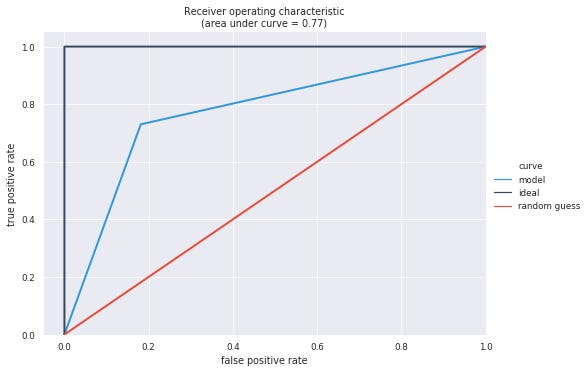Fig 4 — Receiver operating characteristic curve for decomposable attention model

### DEEP LEARNING WITH HIERARCHICAL ATTENTION

This method is adapted from the section Example 2: Hierarchical Attention Networks for Document Classification from Honnibal’s blog post. It implements the ideas presented in the paper Hierarchical Attention Networks for Document Classification by Zichao Yang et al. This model uses attention to reduce an encoded matrix (representing a sequence of words) to a vector. It does this by learning context vectors for two attention stages (one for words and the other for questions).

The attention steps can be seen as a feature extraction process which can be used to learn the similarity between words and between questions.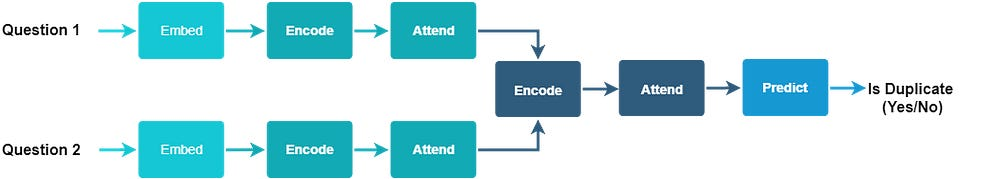High-level components of the neural network. This model encodes and applies attention to each question individually before encoding and applying attention to the combined question-pair

The layers of the model are:

1. Embed — Converts tokens from text to vector space.
2. Encode Words — Uses a bidirectional GRU to encode each word into a context-specific vector. For every token vector, a forward GRU encodes the forward hidden state and a backward GRU encodes the backward hidden state. A bidirectional wrapper layer then concatenates the forward and backward hidden states to summarize the information of the whole question centered around the word. Here, GRU is a recurrent neural network that uses a gating mechanism to track the state of sequences without using separate memory cells (sec 2.1 of Yang et al.).
3. Word Attention — Extracts the important words that are representative of the meaning of the question by using attention and computes a single vector for each question by giving higher weights to the more important words.
4. Encode Questions — Uses a bidirectional GRU to encode each question into a context-specific vector. For every question vector, a forward GRU encodes the forward state and a backward GRU encodes the backward hidden state. A bidirectional wrapper layer then concatenates the forward and backward hidden states to summarize the information of the set of questions.
5. Question Attention — Extracts the important features that are representative of the relationship between the two questions by using attention. It computes a single vector for both questions by giving higher weights to the more important features.
6. Predict / Classify — Involves the final multi-layer perceptron (MLP) with sigmoid activation to predict the output.

Overfitting is countered with dropout regularization and early stopping.Attention layer for the hierarchical attention modelFunction to build the hierarchical attention model

#### Hyperparameter Tuning

The hyperparameters of the model are:

1. Dropout regularization rate (word encoder)
2. Dropout regularization rate (question encoder)
3. Optimization method (Adam / RMSProp / Nadam / SGD )
4. Initial learning rate (for the exponential decay)
5. Learning rate decay (for the exponential decay)
6. Number of samples (batch size) per gradient update
7. The number of epochs used for training

The model parameters are optimized using hyperopt. Two optimization searches are performed: a quicker coarse-grained run and a more fine-grained run, building on the results of the first one. The plots below track the variation of training and validation accuracy during the two searches. There are several instances where the model overfits (points at which validation accuracy is significantly lower than the training accuracy).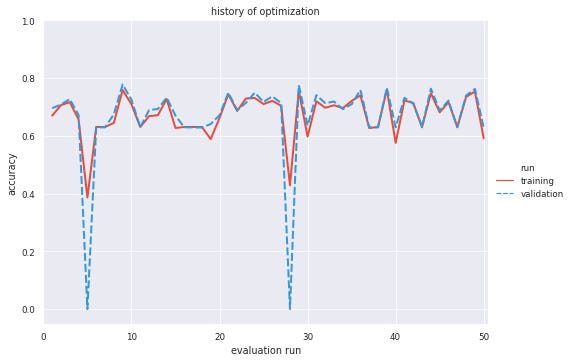The variation of training and validation accuracy during the first hyperparameter search (50 evaluations)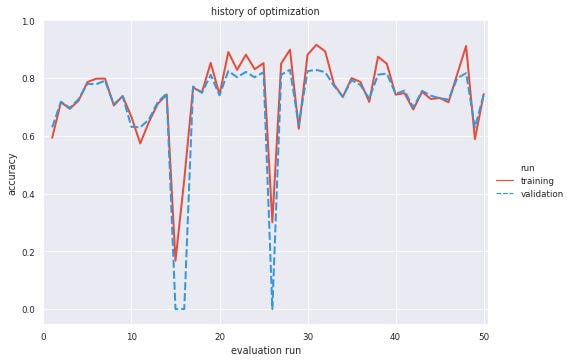The variation of training and validation accuracy during the second hyperparameter search (50 evaluations)Tuning the hierarchical attention model using hyperopt

#### Model Evaluation

The performance of this model is better than the decomposable attention method and at par with the gradient boosted machine.

Model evaluation metrics computed on the test data are as follows:

`accuracy ............... 0.83`
`precision .............. 0.76`
`recall ................. 0.79`
`area-under-roc-curve ... 0.83`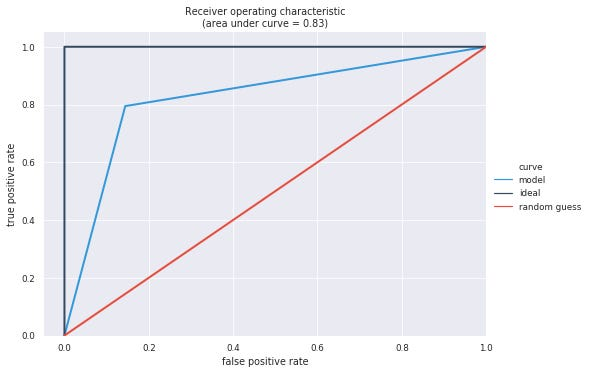Receiver operating characteristic curve for hierarchical attention model

### CONCLUSION AND FURTHER EXPLORATION

The model evaluation results indicate that gradient boosted machine and deep learning with hierarchical attention are effective ways of solving the problem of duplicate identification.

Further exploration should involve the use of alternative neural network architectures and different text preprocessing and embedding techniques.

### REFERENCES

#### Deep Learning

Huge thanks to my Springboard mentor Srdjan Santic for his guidance with this project.